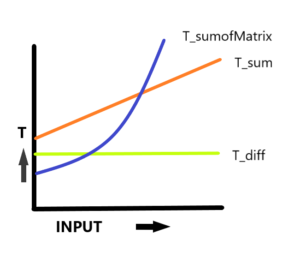# How to Calculate Running Time of an Algorithm?

In this article, we will learn how to deduce and calculate the Running Time of an Algorithm. Also, we will see how to analyze the Time Complexity of the Algorithm. This is very useful when it comes to analyzing the efficiency of our solution. It provides us with the insight to develop better solutions for problems to work on.

Now, the Running Time of an Algorithm may depend on a number of factors :

1. Whether the machine is a Single or Multiple Processor Machine.
2. It also depends on the cost of each Read/Write operation to Memory.
3. The configuration of machine – 32 bit or 64 bit Architecture.
4. The Size of Input given to the Algorithm.

But, when we talk about the Time Complexity of Algorithm we do not consider the first 3 factors. We are concerned with the last factor i.e. how our program behaves on different Input Sizes. So, mostly we consider the Rate of Growth of Time with respect to the input given to the program.

Now, to determine the Run time of our program, we define a Hypothetical Machine with the following characteristics: Single Processor, 32 bit Architecture. It executes instructions sequentially. We assume the machine takes 1 Unit of Time for each operation ( E.g. Arithmetical, Logical , Assignment, Return etc.).

We take a few examples and try to deduce the Rate of Growth with respect to the input.

Let’s say we have to write a program to find difference of two integers.

Explanation:

This is the Pseudocode, if we run this program using the model Machine we defined, the total time taken is Tdiff = 1+1+1 =3 units. So we say irrespective of the size of inputs the time taken for execution is always 3 units or constant for every input. Hence, this a Constant Time Algorithm. So, Rate of Growth is a Constant function. To indicate the upper bound on the growth of algorithm we use Big-O Asymptotic Notation. So, to simplify time complexity is O(1) or constant time because the operations only happen once. Since each of our operations has a runtime of O(1), the Big O of our algorithm is O(1 + 1 + 1) = O(3), which we will then simplify to O(1) as we strip our constants and identify our highest-order term. Hence, the Running time will be O(1) .

Let us look at another example suppose we need to calculate the sum of elements in a list.

Explanation:

This is the Pseudocode for getting the sum of elements in a list or array. The total time taken for this algorithm will be the Cost of each operation * No. of times its executed. So,  Tsum = 1 + 2 * (N+1) + 2* N + 1 = 4N + 4 .

The constants are not important to determine the running time. So, we see the Rate of Growth is a Linear Function, since it is proportional to N, size of array/list. So to simplify the running time and considering the highest order term we say the Running Time is is : O(N) .

Now, if we have to calculate the sum of elements in the matrix of size N*N. The Pseudocode looks like this.

Explanation:

The 1st for loop executes N+1 times for each row to reach end condition (i=n), the 2nd for loop executes (N+1) * (N+1) times for each cell in a column. So, the total time taken by the algorithm,

TsumOfMatrix = 1 + 2 * (N + 1) + 2 * (N+1) * (N+1) + 2 * N * N + 1 = 9N2 + 6N +6.

So on ignoring the lower order terms and constant we see the Rate of Growth of Algorithm is a Quadratic Function. It is proportional to N2 or the Size of the Matrix. If we plot a graph for the above three functions, for the time taken with respect to its inputs we see:The Tdiff graph is constant, Tsum grows linearly with input n and TsumOfMatrix grows as a Square Function giving a Parabolic graph. So, in general, we say Running Time of Algorithm = Σ Running Time of All Fragments of Code.

That’s it for the article, you can try out various examples and follow the general thumb rule discussed to analyze the Time Complexity.

1.